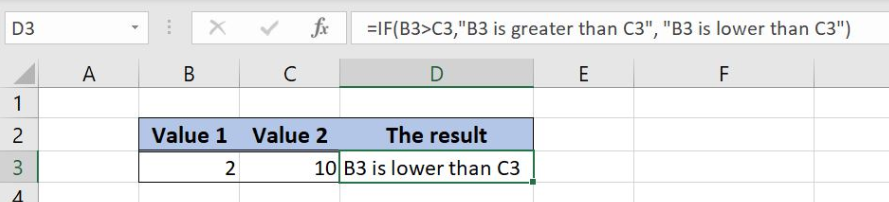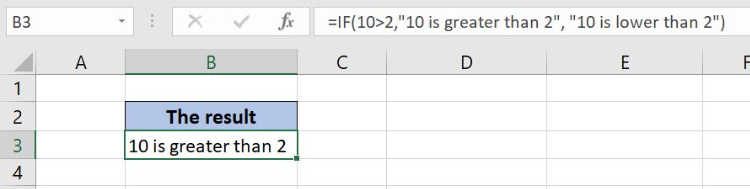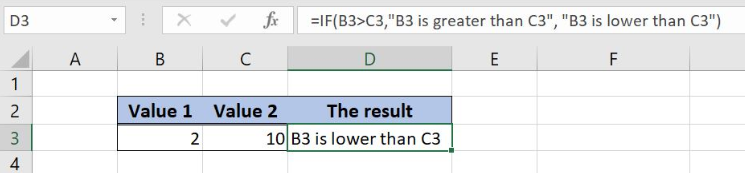Get instant live expert help with Excel or Google Sheets“My Excelchat expert helped me in less than 20 minutes, saving me what would have been 5 hours of work!”

#### Post your problem and you’ll get Expert help in seconds.

Your message must be at least 40 characters
Our professional Expert are available now. Your privacy is guaranteed.

# Excel IF Function

Excel allows a user to use a logical expression in order to check if a condition is true. This is possible by using the IF function which returns Boolean TRUE or FALSE as a result. This step by step tutorial will assist all levels of Excel users in using the IF function.Figure 1. The result of the IF function

## Syntax of the IF Formula

The generic formula for the IF function is:

`=IF(logical_test, value_if_true, value_if_false)`

The parameters of the IF function are:

• logical_test – a logical expression that we want to check
• value_if_true – a value which the function returns if a logical_test is TRUE
• value_if_false – a value which the function returns if a logical_test is FALSE.

## Compare Two Numbers Using the IF Function

In our example, we want to get the check if 10 is greater than 2. In case this is true, we return the message “10 is greater than 2”, and if it’s lower “10 is lower than 2”.

The formula looks like:

`=IF(10>2, "10 is greater than 2", "10 is lower than 2")`

The parameter logical_test is 10>2, as we want to check if 10 is greater than 2. The value_if_true is “10 is greater than 2”, while the value_if_false is “10 is lower than 2”.

To apply the IF function, we need to follow these steps:

• Select cell B3 and click on it
• Insert the formula: `=IF(10>2, "10 is greater than 2", "10 is lower than 2")`
• Press enterFigure 2. Using the IF function to compare two numbers

The logical_test 10>2 is TRUE, so the IF function returns value_if_true. Therefore, the result in the cell B3 is “10 is greater than 2”.

## Using the IF Function to Compare Two Cells

In this example, we will provide cells references to the function, instead of numbers. The example is similar, but now we put 2 in B3 and 10 in C3. The logical_test is now B3>C3. The value_if_true is “B3 is greater than C3”, while the value_if_false is “B3 is lower than C3”. In the cell D3, we want to get the result.

The formula is:

`=IF(B3>C3,"B3 is greater than C3", "B3 is lower than C3")`

To apply the IF function, we need to follow these steps:

• Select cell D3 and click on it
• Insert the formula: `=IF(B3>C3,"B3 is greater than C3", "B3 is lower than C3")`
• Press enterFigure 3. Using the IF function to compare two cells

Now the logical condition is FALSE since 2 is not greater than 10. Therefore, the result in the cell B3 is “B3 is lower than C3”.

Most of the time, the problem you will need to solve will be more complex than a simple application of a formula or function. If you want to save hours of research and frustration, try our live Excelchat service! Our Excel Experts are available 24/7 to answer any Excel question you may have. We guarantee a connection within 30 seconds and a customized solution within 20 minutes.

### Did this post not answer your question? Get a solution from connecting with the expert.Another blog reader asked this question today on Excelchat:
Solution examplesCould you help me by explaining the IF function?
Solved by G. L. in 24 minsI have a question about Conditional Formatting. =IF('Sheet1'!A1="X",IF('Sheet1'!B1="Y", TRUE, FALSE),FALSE) the set the cell background to red. I would like to make it so that if Sheet1'!B1="Z" I set the back to blue. How can I achieve this.
Solved by B. F. in 39 minsI need to find out if the 2018 yearly sales goals were met if the yearly sales were \$25,000 or more using an IF logical function and to set the formula to return a value of YES if met, and NO if not
Solved by A. A. in 18 minsI filtered data in column A (it is labeled ID #) of a data set. On my next worksheet, column A is also the ID #, but it is a different data set. I want to filter the ID #'s the same for the two sheets, but how do I do that being that I am working with two different sets of data?
Solved by V. H. in 22 minsi want to create a very simple if function in a cell which is, if one cell is less than 1250, then the automatic value in the current cell is 250, dead easy, for someone who knows! Thank you.
Solved by A. H. in 14 mins## Subscribe to Excelchat.coAnother blog reader asked this question today on Excelchat: